# 7th Grade - Area of Triangles

## Introduction

• A triangle is a closed polygon formed by three line segments (sides).
• It has three sides, three angles, and three vertices.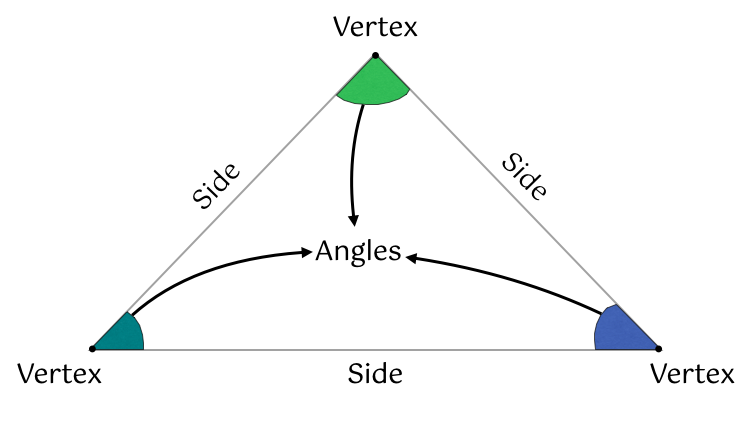## Area of Triangles

• The area of a triangle is the region enclosed by its three sides.
• The area of different triangles differs based on their size.
• If we know the base’s length and the triangle’s height, we can determine its area using the formula mentioned below.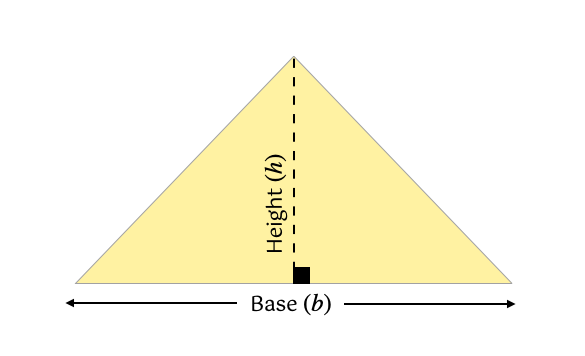, where $b=$length of the base, and $h=$height of the triangle

## Solved Examples

Example 1: Find the area of the triangle shown below.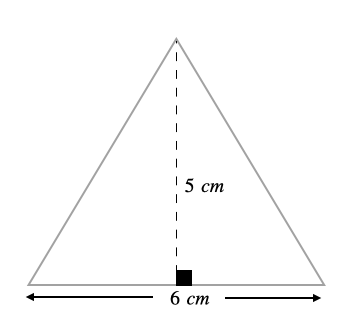Solution: ${A}_{triangle}=\frac{1}{2}×b×h$ $=\frac{1}{2}×6×5$

Example 2: Find the area of the triangle shown below.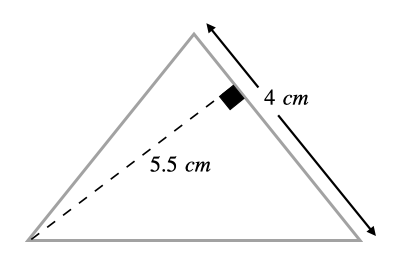Solution: ${A}_{triangle}=\frac{1}{2}×b×h$ $=\frac{1}{2}×4×5.5$

Example 3: Find the area of the triangle shown below.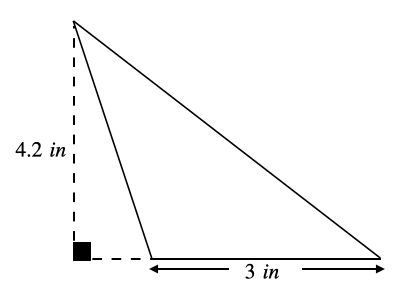Solution: ${A}_{triangle}=\frac{1}{2}×b×h$ $=\frac{1}{2}×4×5.5$

Example 4: Find the area of the triangle shown below.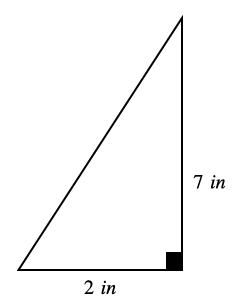Solution: ${A}_{triangle}=\frac{1}{2}×b×h$ $=\frac{1}{2}×2×7$

## Cheat Sheet

• $Are{a}_{triangle}=\frac{1}{2}×b×h$, where $b=$length of the base, and $h=$height of the triangle

## Blunder Areas

• It is vital to identify a triangle’s base and perpendicular height.